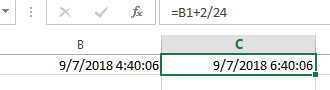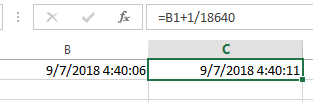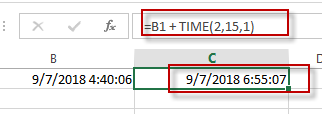# Adding Hours, Minutes, or Seconds to a Date and Time in Excel

This post will guide you how to add hours, minutes, or seconds to a given date and time in a cell in Excel. How do I add hours, minutes or seconds to a time with a formula in Excel. How to add hours to a date and time in Excel. How do I add 15 minutes to a time in excel.

## Add Hours to a Date and Time

If you want to add 2 hours to a specific date and time in one cell, you can use the following formula to achieve the result.

`=B1+ 2/24 `

You need to type this formula into a blank cell and then press Enter key in your keyboard, and drag the AutoFill Handle over other cells to apply this formula to add 2 hours for other dates.This formula will return a serial number, so you need to format the serial number as the standard date and time format.

## Add Minutes to a Date and Time

If you want to add 15 minutes to a date and time in one cell, you can use the following formula:

`=B1 + 15/1440`

## Add Seconds to a Date and Time

If you want to add 1 second to a date and time in one cell, you can use the following formula:

`=B1 + 1/18640`You can also create another formula based on the TIME function to achieve the same result.
For example, you want to add 2 hours, 15 minutes, 1 second to a data and time value in Cell B1, you can use the following formula:

`=B1 + TIME(2,15,1)`Related Posts

Calculate Grades With VLookup in Excel

Why Should You Calculate Grades With VLookup Excel? If you're looking for a simple way to find out a student's grades, VLOOKUP Excel can do the trick. This function uses a lookup table to find the values and sort them ...

Repeating Character n Times in Excel

In daily life, we can use repeated characters to indicate the magnitude or priority of something. For example, a single “!” indicates a minor case, double “!!” indicates a medium case, and triple “!!!” indicates a high case, and so ...

Calculate Cumulative Loan Interest in Excel

What is Cumulative Loan Interest? When comparing different types of loans, many people want to know what is Cumulative Loan Interest. The sum of all interest payments you've made on a loan is referred to as cumulative interest. Different lenders ...

Calculate Cumulative Loan Principal Payments in Excel

How do you Calculate Cumulative Loan Principal Payments in Excel? There are a few different ways to calculate this information, but one way has been proven to be accurate more often than others. One option is to use the CUMPRINC ...

Trap Error or Replace Error by Specific Value with IFERROR function

We often use Excel formulas in our work life, and we may encounter this situation that the formula throws an error and finally an error like #DIV/0! Is displayed in the cell. In today’s tutorial, we will introduce you the ...

Sidebar